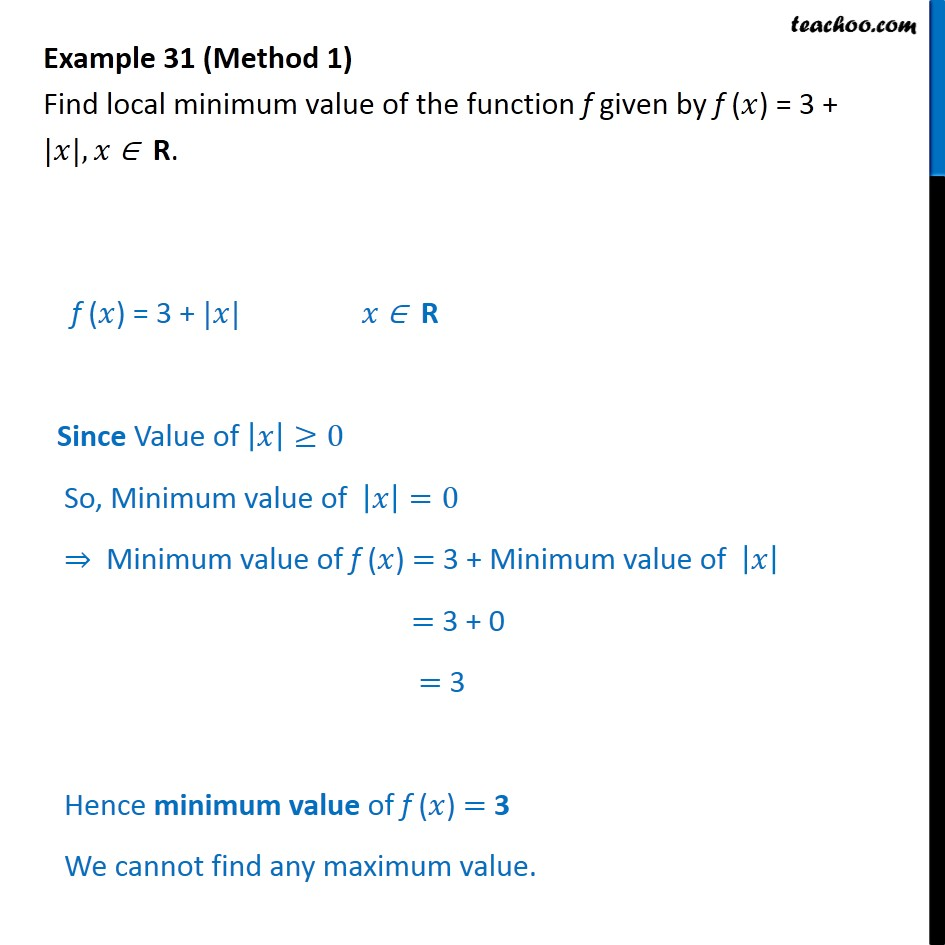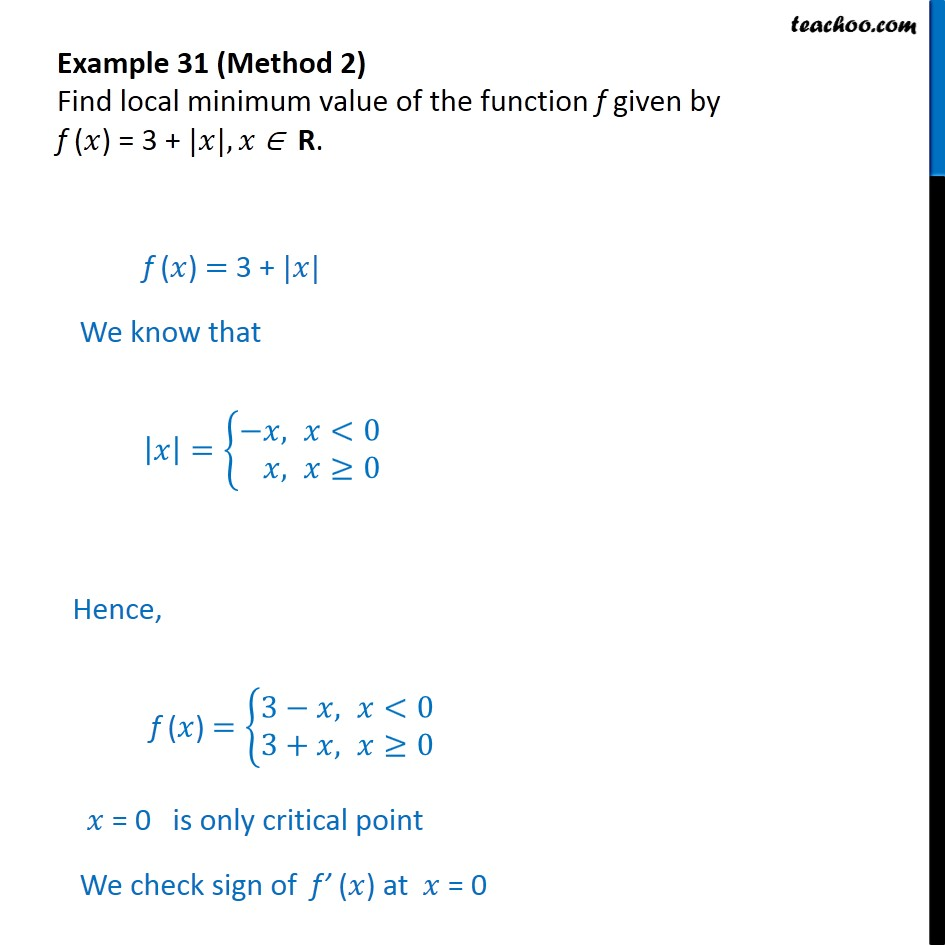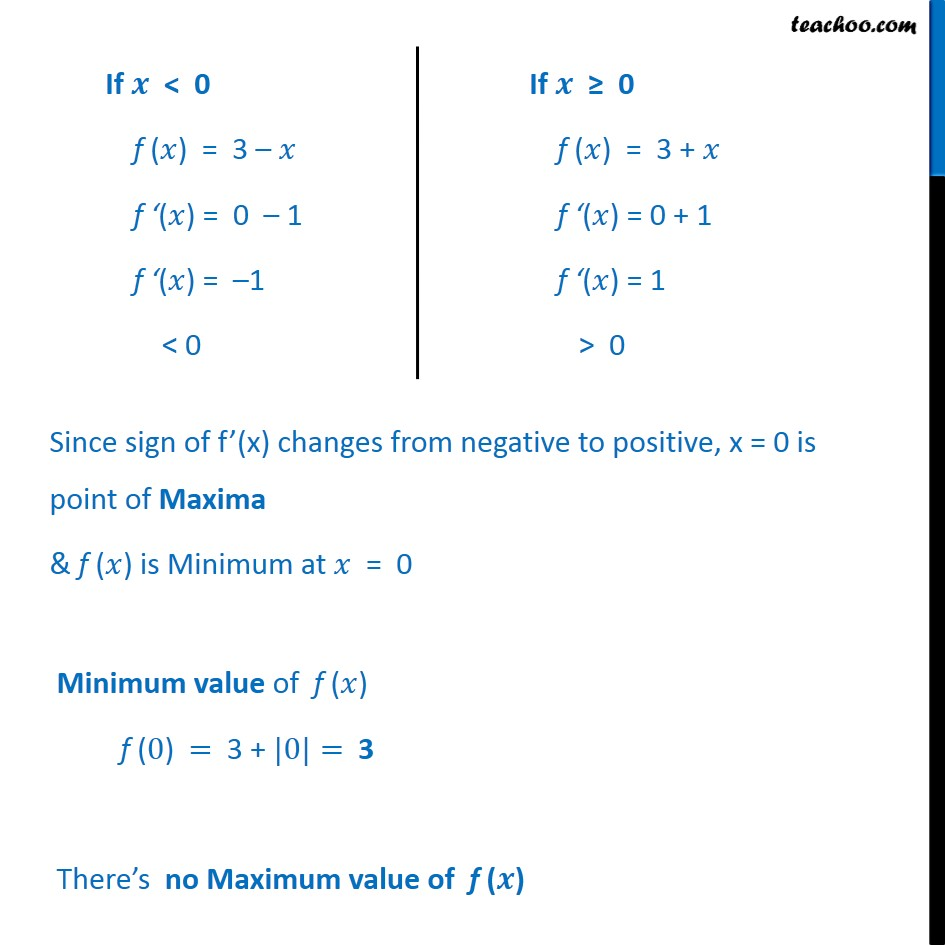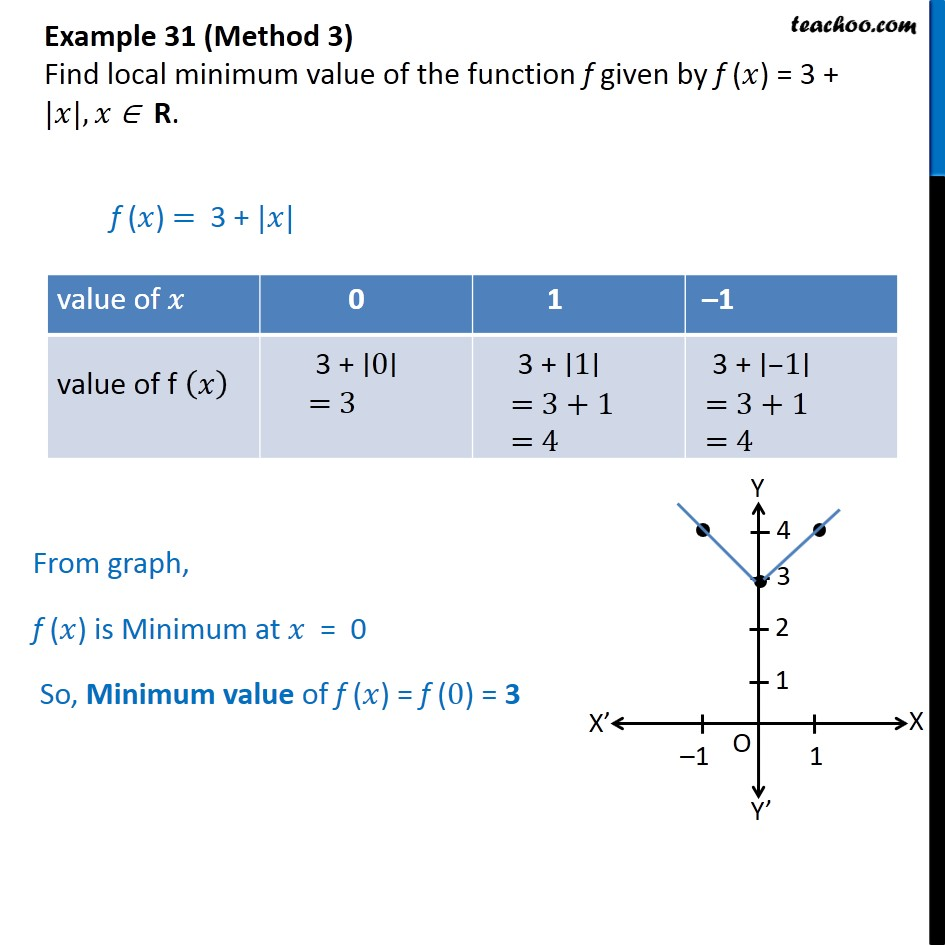1. Chapter 6 Class 12 Application of Derivatives
2. Serial order wise
3. Examples

Transcript

Example 31 (Method 1) Find local minimum value of the function f given by f ( ) = 3 + | |, R. f ( ) = 3 + | | R Since Value of 0 So, Minimum value of =0 Minimum value of f ( ) = 3 + Minimum value of = 3 + 0 = 3 Hence minimum value of f ( ) = 3 We cannot find any maximum value. Example 31 (Method 2) Find local minimum value of the function f given by f ( ) = 3 + | |, R. f ( ) = 3 + | | We know that = & , <0 & , 0 Hence, f ( ) = 3 & , <0 3+ , 0 = 0 is only critical point We check sign of f ( ) at = 0 Since sign of f (x) changes from negative to positive, x = 0 is point of Maxima & f ( ) is Minimum at = 0 Minimum value of f ( ) f (0) = 3 + |0|= 3 There s no Maximum value of f ( ) Example 31 (Method 3) Find local minimum value of the function f given by f ( ) = 3 + | |, R. f ( ) = 3 + | | From graph, f ( ) is Minimum at = 0 So, Minimum value of f ( ) = f (0) = 3

Examples